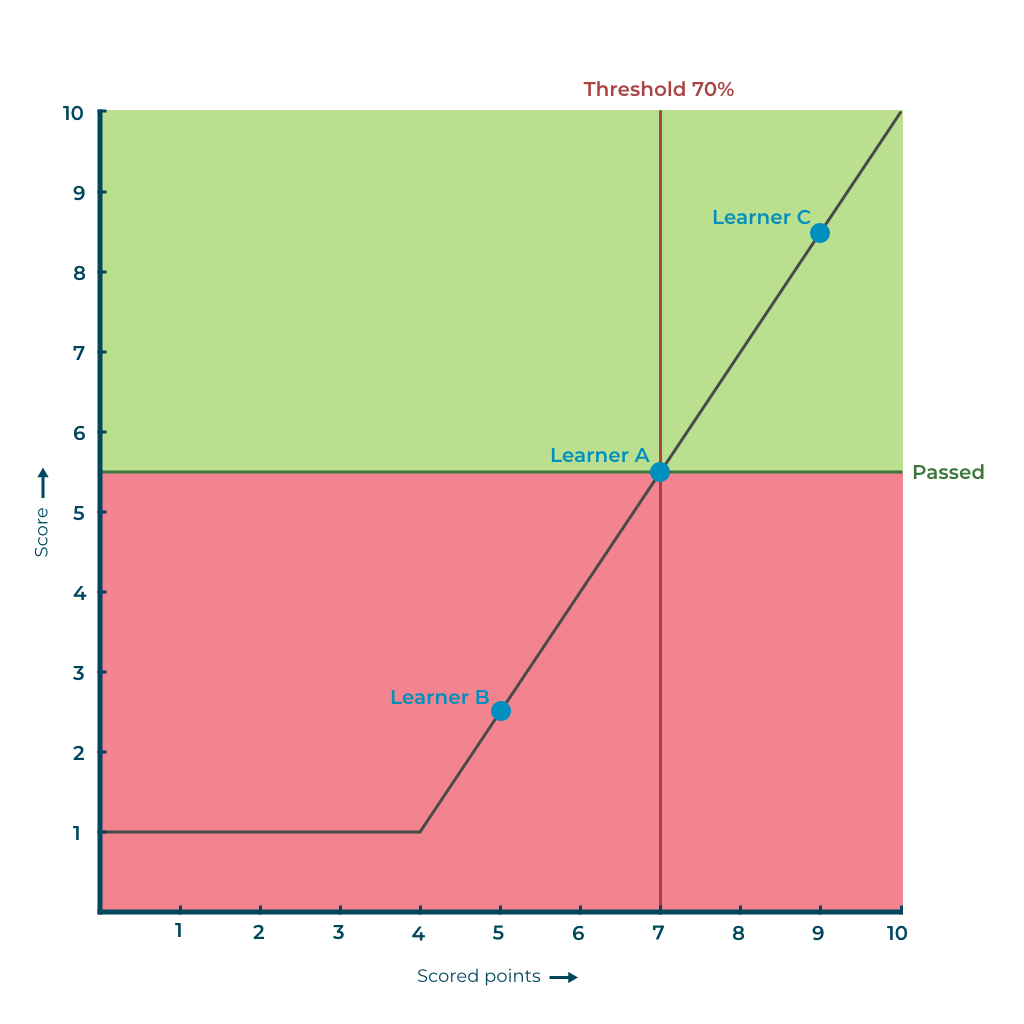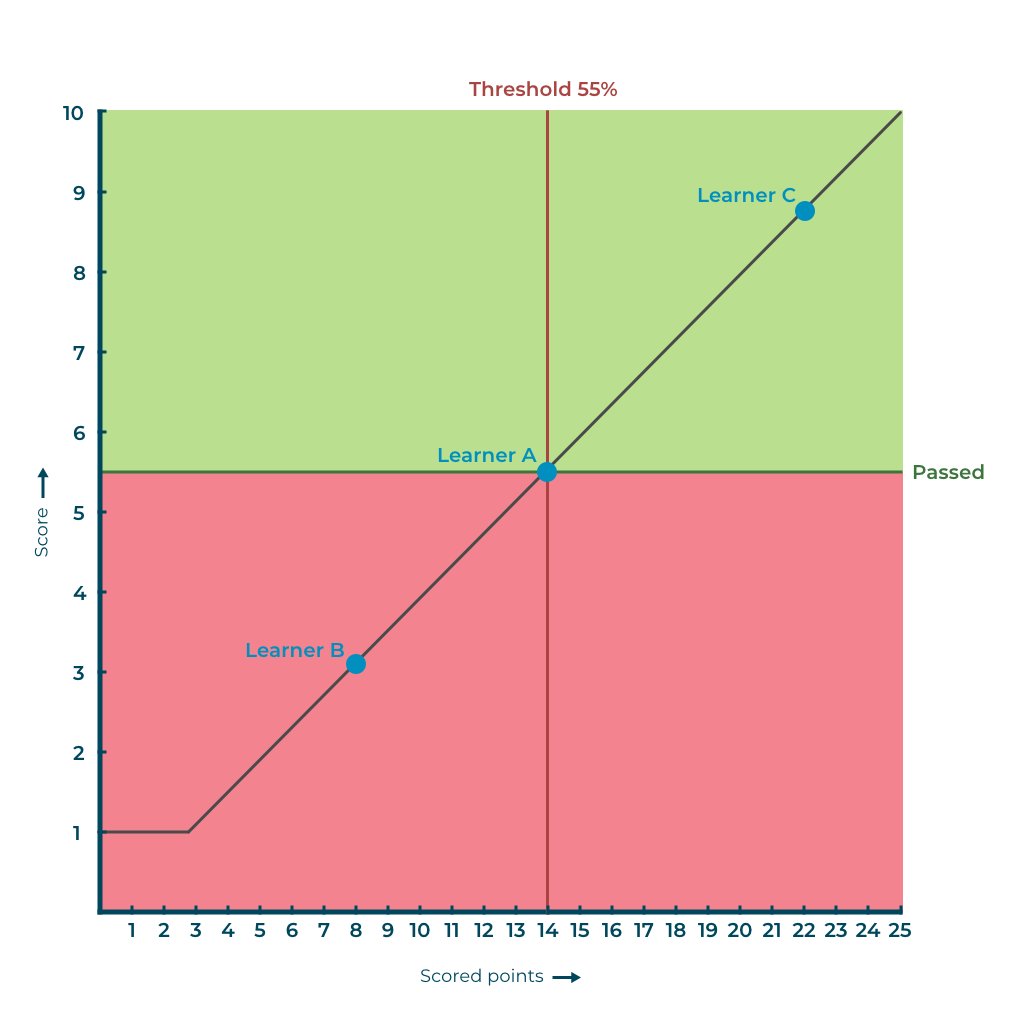By default, the score of an assessment is displayed and calculated as a percentage. If you want the score to be displayed on a scale of 1 to 10, it is not always easy to display the right score to learners.

aNewSpring sees a score of 5.5 as a threshold. Is it the norm for you that a learner should pass at a different score, like a 5 or 6? Then have the score calculated based on percentages instead of numbers.

We will explain how the threshold works in relation to the number of questions with a few examples.

You can set the score you get per question, but for the sake of convenience, each question is worth 1 point in the examples below.

##### Example 1

Number of points to be achieved: 10
Threshold: 70%
Rounding to: Decimals

Learner A answered 7 questions correctly. That is exactly the 70% required to pass the test. This will be displayed as passed with a score of 5.5.

Learner B answered 5 questions correctly. That is 50% of the total points the learner could get. This is shown as failed with a score of 2.5.

Learner C answered 9 questions correctly. This learner passed with a score of 8.5.The system assumes that you can never score lower than a 1, that is the bottom limit. In this example, someone with 4 points would still get a 1.

##### Example 2

Number of points to be achieved: 25
Threshold: 55%
Round to: Decimals

Learner A answered 14 questions correctly. That is 56% of the total points the learner could get. This is enough to pass the test, so this is shown as passed with a score of 5.5.

Learner B only answered 8 questions correctly and scored 3.2.

Learner C answered 22 questions correctly. This is 88% of the total number of questions, which is a score of 8.8.#### Calculation tool

To make it easy for you, we have made a calculation tool in the form of an Excel file:

calculation-tool-threshold-percentage-score.xlsx

Enter the total number of points that can be scored and the threshold as a percentage. In the table you will be able to see the score that learners will see for the number of points they score.

If you work with 'traditional' figures, it is best to have a threshold of 55%. For 55% of 10 questions you will get a 5.5.